# 6.5 Equilibrium output and the AD curve

$$\newcommand{\vecs}{\overset { \rightharpoonup} {\mathbf{#1}} }$$ $$\newcommand{\vecd}{\overset{-\!-\!\rightharpoonup}{\vphantom{a}\smash {#1}}}$$$$\newcommand{\id}{\mathrm{id}}$$ $$\newcommand{\Span}{\mathrm{span}}$$ $$\newcommand{\kernel}{\mathrm{null}\,}$$ $$\newcommand{\range}{\mathrm{range}\,}$$ $$\newcommand{\RealPart}{\mathrm{Re}}$$ $$\newcommand{\ImaginaryPart}{\mathrm{Im}}$$ $$\newcommand{\Argument}{\mathrm{Arg}}$$ $$\newcommand{\norm}{\| #1 \|}$$ $$\newcommand{\inner}{\langle #1, #2 \rangle}$$ $$\newcommand{\Span}{\mathrm{span}}$$ $$\newcommand{\id}{\mathrm{id}}$$ $$\newcommand{\Span}{\mathrm{span}}$$ $$\newcommand{\kernel}{\mathrm{null}\,}$$ $$\newcommand{\range}{\mathrm{range}\,}$$ $$\newcommand{\RealPart}{\mathrm{Re}}$$ $$\newcommand{\ImaginaryPart}{\mathrm{Im}}$$ $$\newcommand{\Argument}{\mathrm{Arg}}$$ $$\newcommand{\norm}{\| #1 \|}$$ $$\newcommand{\inner}{\langle #1, #2 \rangle}$$ $$\newcommand{\Span}{\mathrm{span}}$$$$\newcommand{\AA}{\unicode[.8,0]{x212B}}$$

In Chapter 5 and at the beginning of this chapter, we used an aggregate demand and aggregate supply model to explain business cycle fluctuations in real GDP and employment. In this chapter we have developed a basic explanation for the shifts in AD that cause changes in real output. In the short run:

• wages, prices, money supply, interest rates and exchange rates are assumed to be constant;
• distinction between autonomous and induced expenditures is important; and
• equilibrium real GDP requires output equal to planned aggregate expenditure.
Figure 6.9 Equilibrium GDP and aggregate demandEquilibrium in a) determined the position of the AD curve in b). A change in autonomous expenditure in a) changes equilibrium Y and shifts AD by the change in autonomous expenditure times the multiplier.

In this model, investment and exports are the main sources of fluctuations in autonomous expenditures. The marginal propensities to consume and import describe the changes in aggregate expenditure caused by changes in income. These induced expenditures are the source of the multiplier. When business changes its investment plans in response to predictions and expectations about future markets and profits, or exports change in response to international trade conditions, the multiplier translates these changes in autonomous expenditure into shifts in the AD curve. Shifts in the AD curve cause changes in equilibrium output and employment.

Figure 6.9 shows how this works. Equilibrium real GDP in the upper panel determines the position of the AD curve in the lower panel.

Initially, equilibrium real GDP at the price level P0 is determined by the equilibrium condition Ye=A0+(cm)Y in the upper panel and by the equilibrium conditionin the lower panel.

Changes in autonomous expenditure shift the AD curve. If autonomous expenditure increased from A0 to A1 as shown in panel (a), equilibrium output would increase from Y0 to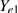. The change in equilibrium output would be (). The AD curve would shift to the right to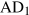as a result of the increase in autonomous expenditure. The size of the horizontal shift would be ().

This model provides an important first insight into the sources of business cycles in the economy. However, it is a pure private household/private business sector economy. Autonomous consumption, investment, exports and imports, and the multiplier drive real GDP and income and fluctuations in those measures of economic activity. There is no government, and thus no way for government policy to affect real output and employment. There is no financial sector to explain the interest rates and foreign exchange rates that affect expenditure decisions, and thus no monetary policy. In the next few chapters we extend our discussion of aggregate expenditure and aggregate demand to include the government sector and financial sectors, as well as fiscal and monetary policy. The framework becomes a bit more complicated and realistic, but the basic mechanics are still those we have developed in this chapter.

Nonetheless, this basic model explains why, in the first half of 2015 both Steven Poloz, the Governor of the Bank of Canada and Joe Oliver, the Minister of Finance are both counting on a strong rise in exports to move the Canadian economy from a recession to growth in the second and third quarters of 2015.

Figure 6.5 above shows that export growth has not provided the hoped for increase in aggregate demand nor did investment expenditures rise to add to aggregate demand. Recognizing these weak economic conditions the new Liberal government Minister of Finance, Bill Morneau, initiated an expansionary fiscal policy program. That policy planned for government budget deficits to finance expenditures on major infrastructure to increase the government component of aggregate demand and stimulate growth in real GDP.

Example Box 6.1 The algebra of the basic income – expenditure model.

The basic model has two components:

1. Aggregate expenditure = Autonomous expenditure plus induced expenditure: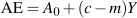(6.2)
• Equilibrium condition: GDP = Aggregate expenditure• Then equilibrium Y is found by substituting 1 into 2:

 Y =A0+(c–m)Y Y–(c–m)Y =A0=A0 YRecall that (cm) is the slope of the AE function (i.e.). Then in equilibrium:Using the numbers in the example in Table 6.3and for equilibrium:

 Y =100+0.5Y Y–0.5Y =100 Y =100/(1–0.5) Y =200
Example Box 6.2 The multiplier in a basic algebraic model.

Initial conditions in a basic model: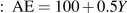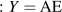Then equilibrium national income is:

 Y =100+0.5Y 0.5Y =100 Y =200

Suppose autonomous expenditure increases by 25 to A1=125:Then the new equilibrium national income is:

 Y =125+0.5Y 0.5Y =125 Y1 =250

The change in autonomous expenditure by 25 increased equilibrium national income by 50.

The Multiplier is defined as the change in national incomedivided by the change in autonomous expenditure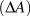that caused it. In this example the multiplier is: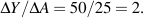Notice the multiplier is also equal to. In this example the slope of AE is the marginal propensity to spend on domestic output (cm)=0.5. The multiplier is:This page titled 6.5 Equilibrium output and the AD curve is shared under a CC BY-NC-SA license and was authored, remixed, and/or curated by Douglas Curtis and Ian Irvine (Lyryx) .